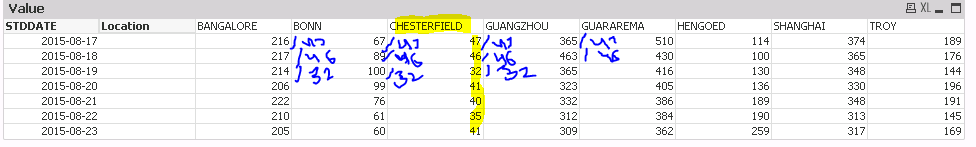# QlikView Scripting

Discussion Board for collaboration on QlikView Scripting.

Announcements

#### Breathe easy -- you now have more time to plan your next steps with Qlik! QlikView 11.2 Extended Support is now valid through December 31, 2020. Click here for more information.

Honored Contributor III

## Pivot table Calculation Help

Hi Folks ,

I have a pivot table :

Dimmension: STDDATE , Location

Expression : Sum(Values)Requirement : I need to divide "CHESTERFIELD" values with other location row wise.

e.g for STDDATE- 2015-08-17 . the entire row need to be divide by 47 , similarly for 2015-08-18 row should be divided by 46 and so on...

Kindly suggest how this can be achieved .

Thanks,

AS

1 Solution

Accepted SolutionsNot applicable

## Re: Pivot table Calculation Help

Hi. In expression you mast write

if(Location<>'CHESTERFIELD',sum({<Location-={CHESTERFIELD}>} Value)/sum(TOTAL<STDDATE> {<Location={CHESTERFIELD}>} Value) ,sum(Value) )

14 Replies
Honored Contributor III

## Re: Pivot table Calculation Help

I read Before and After functions which works only for Pivot table few days back, will give a try now.MVP

## Re: Pivot table Calculation Help

Can you provide the sample app?

Honored Contributor III

## Re: Pivot table Calculation Help

Please see the attachment

Thanks,
AS

Honored Contributor III

## Re: Pivot table Calculation Help

I have PE so cannot see your file, please see attached if it can help.

Something like below can work -

If(ColumnNo()=1,Sum(Value)/After(Sum(Value),2),

If(ColumnNo()=2,Sum(Value)/After(Sum(Value),1),

If(ColumnNo()=3,Sum(Value)/Sum(Value),

If(ColumnNo()=4,Sum(Value)/Before(Sum(Value),1)))))

Honored Contributor III

## Re: Pivot table Calculation Help

Digvijay,

This is working till column 4 , cam you help to build this script till coulmn 8.

Thanks,
AS

Honored Contributor III

## Re: Pivot table Calculation Help

Just a sample,

If(ColumnNo()=1,Sum(Value)/After(Sum(Value),2),

If(ColumnNo()=2,Sum(Value)/After(Sum(Value),1),

If(ColumnNo()=3,Sum(Value)/Sum(Value),

If(ColumnNo()=4,Sum(Value)/Before(Sum(Value),1),

If(ColumnNo()=5,Sum(Value)/Before(Sum(Value),2),

If(ColumnNo()=6,Sum(Value)/Before(Sum(Value),3),

If(ColumnNo()=7,Sum(Value)/Before(Sum(Value),4),

If(ColumnNo()=8,Sum(Value)/Before(Sum(Value),5),

))))))))

I had to change sorting of Location to load order. Also attaching the data I have used.Not applicable

## Re: Pivot table Calculation Help

Hi. In expression you mast write

if(Location<>'CHESTERFIELD',sum({<Location-={CHESTERFIELD}>} Value)/sum(TOTAL<STDDATE> {<Location={CHESTERFIELD}>} Value) ,sum(Value) )

Honored Contributor III

## Re: Pivot table Calculation Help

Great! I feel this is better and dynamic solution. I just checked it is working perfectly on the data I used.Thanks!Not applicable

## Re: Pivot table Calculation Help

Hi Amit,

Try the following:

Sum(Values)/ Sum(Total <STDDATE> {<Location={"CHESTERFIELD"}>} Values)